# Run charts with R

## Introduction

Plotting data over time is a simple method to learn from trends, patterns, and variation in data and to study the effect of improvement efforts.

A run chart is a simple line graph of a measure over time with the median shown as a horizontal line dividing the data points so that half of the points are above the median and half are below.

library(qicharts)
set.seed(9)         # Lock random number generator
y <- rpois(24, 16)  # Random values from to plot
qic(y)              # Plot run chart of y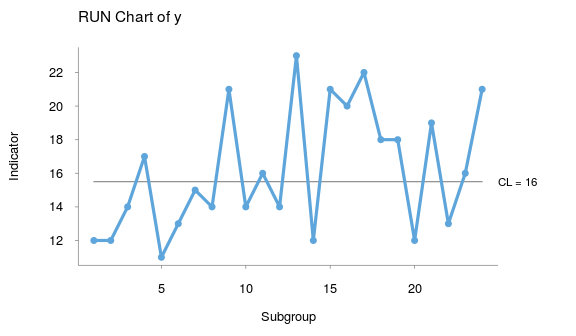Figure 1

The main purpose of the run chart is to detect process improvement or process degradation, which will turn up as non-random patterns in the distribution of data points around the median.

## Testing for non-random variation in run charts

If the process of interest shows only random variation, the data points will be randomly distributed around the median. Random meaning that we cannot know if the next data point will fall above or below the median, but that the probability of each event is 50%, and that the data points are independent. Independence means that the position of one data point does not influence the position of the next data point, that is, data are not auto-correlated.

If the process shifts, these conditions are no longer true and patterns of non-random variation may be detected by statistical tests.

Non-random variation may present itself in several ways. If the process centre is shifting due to improvement or degradation we may observe unusually long runs of consecutive data points on the same side of the median or that the graph crosses the median unusually few times. The length of the longest run and the number of crossings in a random process are predictable within limits and depend on the total number of data points in the run chart (Anhoej 2014, Anhoej 2015).

• A shift signal is present if any run of consecutive data points on the same side of the median is longer than the prediction limit, round(log2(n) + 3). Data points that fall on the median do not count, they do neither break nor contribute to the run (Schilling 2012).

• A crossings signal is present if the number of times the graph crosses the median is smaller than the prediction limit, qbinom(0.05, n - 1, 0.5) (Chen 2010).

n is the number of useful data points, that is, data points that do not fall on the median.

The shift and the crossings signals are based on a false positive signal rate around 5% and have proven useful in practice.

y[13:24] <- rpois(12, 24)  # Introduce a shift in process mean
qic(y)                     # Plot run chart of y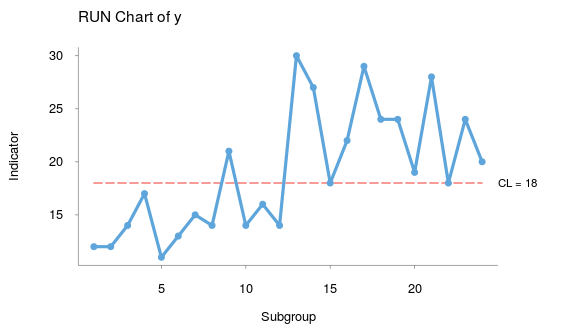Figure 2

Figure 2 show a run chart with 24 data points of which 22 are not on the median. The longest run of consecutive data points on the same side of the median is 10 (not counting the two data points that fall on the median); and the graph crosses the median 3 times. Since the longest run is longer that predicted (7) and the number of crossings is smaller than predicted (7), we may conclude that the process exhibits non-random variation.

The shift and crossings signals are two sides of the same coin and will often signal together. However, any one of them is diagnostic of non-random variation.

Signal limits may be tabulated like this:

n <- 10:30
data.frame(
n.useful      = n,
longest.run   = round(log2(n) + 3),
min.crossings = qbinom(0.05, n - 1, 0.5))
##    n.useful longest.run min.crossings
## 1        10           6             2
## 2        11           6             2
## 3        12           7             3
## 4        13           7             3
## 5        14           7             4
## 6        15           7             4
## 7        16           7             4
## 8        17           7             5
## 9        18           7             5
## 10       19           7             6
## 11       20           7             6
## 12       21           7             6
## 13       22           7             7
## 14       23           8             7
## 15       24           8             8
## 16       25           8             8
## 17       26           8             8
## 18       27           8             9
## 19       28           8             9
## 20       29           8            10
## 21       30           8            10

## Analysis of before-and-after data

If data have been collected before and after a change, it may be useful to calculate the median only from the data points that belong to the before period.

qic(y, freeze = 12)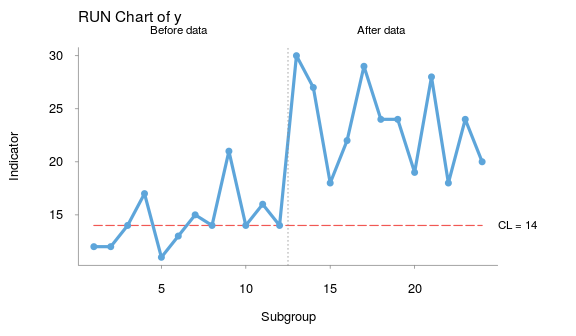Figure 3

If a significant change in process performance has occurred, it may be useful to split the graph in two.

qic(y, breaks = 12)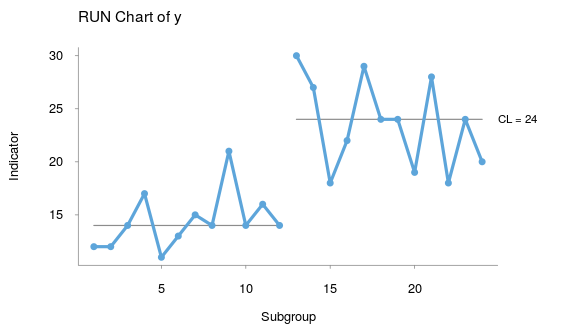Figure 4

## Plotting proportion and rates

If one needs to plot proportions or rates, the denominator may be provided as the second argument, n.

y <- rbinom(24, 20, 0.5)                # Numerator
n <- sample(16:20, 24, replace = TRUE)  # Denominator
qic(y, n)                               # Plot run chart of y/n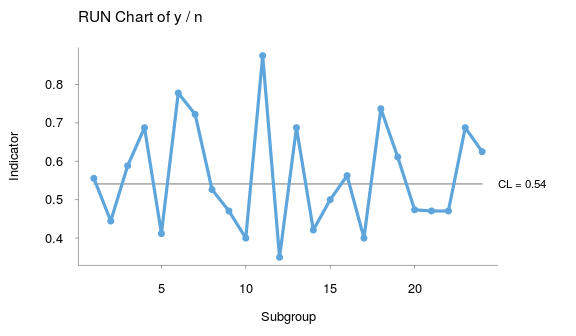Figure 5

## Using title, labels, and annotations

Tick mark labels for the x axis may be provided with the x argument. Chart title and labels for the x and y axis are provided the usual way using the main, xlab, and ylab arguments. Annotations can be added by the notes argument, which takes a character vector containing text to be added to individual data points.

startdate <- as.Date('2014-1-6')
date      <- seq.Date(startdate,         # Dates for x axis labels
by = 'day',
length.out = 24)
notes     <- NA
notes <- 'This is a note'            # Character vector of annotations
qic(y, n,
x     = date,
main  = 'Run Chart',
ylab  = 'Proportion',
xlab  = 'Date',
notes = notes)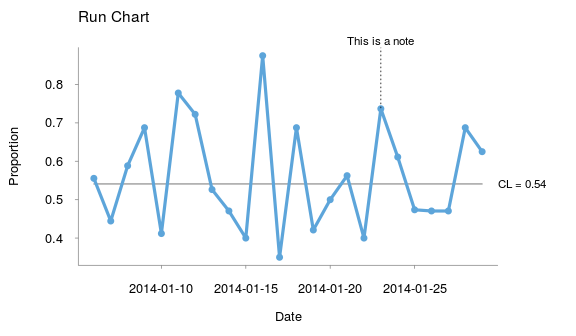Figure 6

## Automatic data aggregation by subgroups

Besides providing tick mark labels for the x axis, the x argument serves as a subgrouping vector. If, for instance, one collects data daily but wishes to aggregate data by week, this can be achieved by using weeks as the subgrouping, x, vector.

This example uses a data frame containing the numerator, the denominator, and the subgroups to plot.

date      <- seq.Date(startdate, by = 'day',       # 20 week long day sequence
length.out = 7 * 20)
n         <- sample(3:5, 7 * 20, replace = TRUE)   # Denominator vector
y         <- rbinom(7 * 20, n, 0.5)                # Numerator vector
week      <- as.Date(cut(date, 'week'))            # Subgrouping vector
d         <- data.frame(date, y, n, week)          # Data frame
head(d, 10)
##          date y n       week
## 1  2014-01-06 3 5 2014-01-06
## 2  2014-01-07 1 4 2014-01-06
## 3  2014-01-08 2 5 2014-01-06
## 4  2014-01-09 3 5 2014-01-06
## 5  2014-01-10 3 5 2014-01-06
## 6  2014-01-11 2 5 2014-01-06
## 7  2014-01-12 2 3 2014-01-06
## 8  2014-01-13 3 5 2014-01-13
## 9  2014-01-14 1 3 2014-01-13
## 10 2014-01-15 3 4 2014-01-13

By using the data argument, we may avoid the clumsy \$ notation. And by using the week column as the subgrouping vector, the qic function takes care of aggregating and plotting data by subgroups.

qic(y, n, x = week, data = d)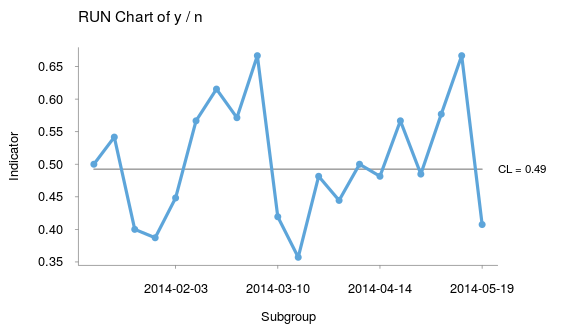Figure 7